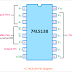IC 74138 is a Logical Decoder IC. It also has a demultiplexing facility. The IC 74138 is available in the market with the name of 74LS138. It is a 3 to 8 decoder IC. The internal circuit of this IC is made of high-speed Schottky barrier diode.

## IC 74138 Pin Diagram

As you see in the below pin diagram, the IC 74LS138 has a total of sixteen pins.

1. The pin no. 8 and 16 are the ground and Vcc respectively for the power input.

2. There are a total of three input pins(pin no. 1, 2, 3). They are denoted by A0, A1, A2. So the IC 74LS138 can take three binary input signals.

3. There are three enable input pins E1, E2, E3(pin no. 4, 5, 6). E1 and E2 are the active LOW pins that mean when low signals are applied to those pins they will be active.

4. Pin no. 7 and 9 to 15 are the output pins.

## Logical Diagram of IC 74138

The logical circuit of the IC 74138 is made using NOT Gate, and AND Gates. You can see the circuit diagram in the below figure.

## IC 74138 Truth Table

Here the truth table of IC 74138 is given below.

## Operating Condition of IC SN74LS138

1. The supply voltage or Vcc should be given between 4.75V to 5.25V
2. Operating temperature range should be between 0 to 70-degree centigrade

## Features of IC 74138

1. It is very fast and high-speed IC.
2. It consumes very low power because it consists of low power Schottky diodes.
3. It has a demultiplexing facility.
4. It has a very short propagation delay.
5. Appropriate operating Temperature.

## IC 74138 Applications

1. It is a decoder IC, the main application is to decode the digital signal.

2. They are used in digital memory circuits.

3. They are used in data routing applications.

4. They are used for demultiplexing of digital signals.

IC 74138 Pin Diagram, Truth Table, Logical Circuit, ApplicationsReviewed by Author on June 27, 2019 Rating: 5